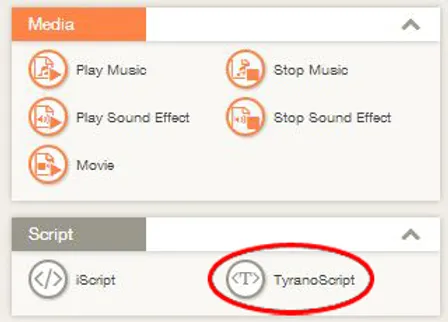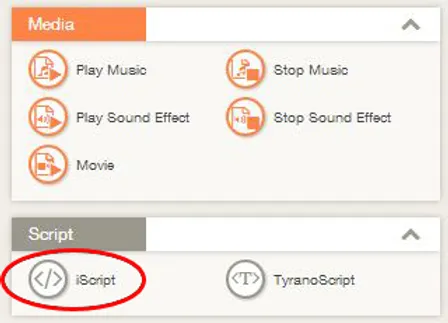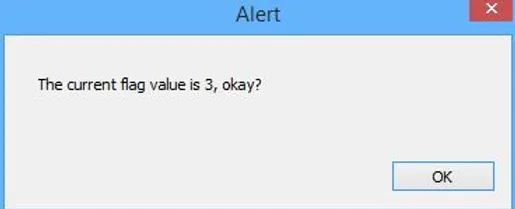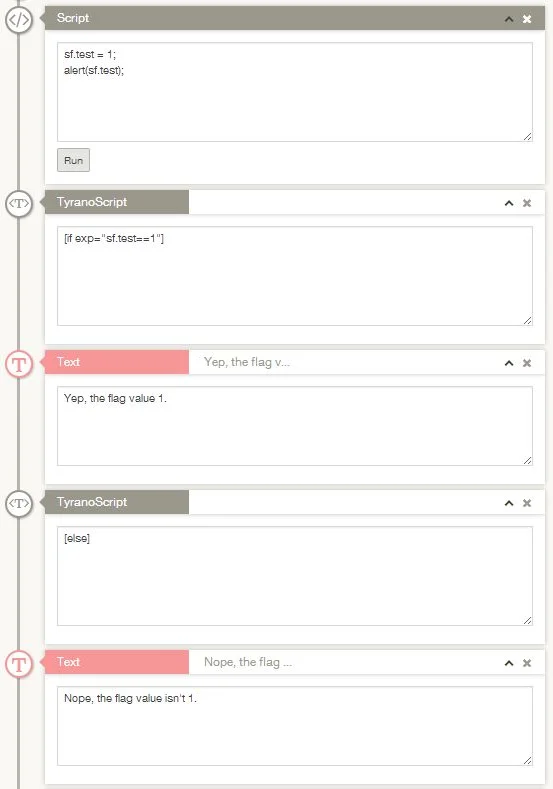# Flags and Variables – Using TyranoScript

TyranoScript is used by inserting the ‘TyranoScript‘ component into the Scene Editor.
From the Tools Area, drag and drop the TyranoScript component into the Scene Editor.The TyranoScript component in the Scene Editor has a field for entering text, right?
This is where we’ll enter the scripting for our flags and variables.

Let’s look at some sample script using variables.

``````
;This assigns a text string to a system variable
[eval exp="sf.variable1 = 'Sample Text'"]

;This assigns a number to a game variable
[eval exp="f.flag1 = 1000"]

;This assigns a temporary variable
[eval exp="tf.flag1 = f.flag2"]
``````

The assigned values can be used in a variety of ways, such as referencing them when the game makes conditional jumps, or displaying them on screen for the player to see.

## Using the iScript Component

The ‘iScript’ component works just like the TyranoScript component, but allows you to use the full power of JavaScript in TyranoBuilder.

It would be no exaggeration to say that JavaScript is probably the most widely used programming language in the world, so by learning to use iScript, you’re actually learning one of the most practical programming languages there is and you can easily put this knowledge to use in other applications!

From the Tools Area, drag and drop the iScript component into the Scene Editor.JavaScript is entered via the text field of the iScript component in the Scene Editor.

You can use the iscript component to chain a series of evals together. For example, the code below has exactly the same effect as the previous TyranoScript code sample.

``````
[iscript]

sf.variable1 = 'Sample Text'
f.flag1 = 1000
tf.flag1 = f.flag2

[endscript]
``````

Let’s look another example. Type the below code into the iScript text area:

``````
var flag = 3;
alert("The current flag value is "+flag+", okay?");
flag = flag + 3 ;
alert("Now the flag value is "+flag+". Sweet!");
``````

Save the project and preview the game.
If you see two pop-ups like below appear, then bingo!You get the idea, right?
In the first line, var flag=3 prepares the variable ‘flag’ and gives it the value 3. Then, the flag value is displayed using alert().
In the next line, flag = flag + 3 adds another 3 to the value of flag.

## Naming Variables

TyranoScript allows you name variables as you like, with the single rule that a variable cannot begin with a number. E.g. f.3name cannot be used as a name for a variable.

## Using Variables to Affect Game Progression

Let’s say you want to have a particular event happen or different story branches followed depending on the value of the flags and variables you set. This is done by using TyranoScript’s if statements which we’ll cover right now.

Here’s an example of using iScript to set a variable and then using TyranoScript to evaluate the value and execute different component accordingly.To distinguish between providing a good ending or bad ending, all you need to do is add or subtract variable values depending on the choices that players make or the route they follow during the game. Then, check the variable values before the ending, and jump to the good ending or bad ending depending on the value.

We’ll look at how to perform calculations with variables and how to evaluate the results in the next couple of sections.

## Performing Calculations with Variables

Right then, let’s look at how to perform calculations with variables.

``````
[eval exp="f.flag1 = f.flag1 + 1"]
``````

In the above example, we added 1 to the value of f.flag1 and stored the result in the f.flag1 variable. (In other words, we’ve added 1 to f.flag1.)

``````
[eval exp="f.flag1 = f.flag1 + f.flag2 * f.flag3"]
``````

This time, the result of f.flag2 multiplied by f.flag3 + f.flag1 is stored in f.flag1.

To divide, use slash (/).

You can also use + to concatenate strings like this:

``````
[eval exp="f.flag2 = 'sticky '"]
[eval exp="f.flag3 = 'toffee'"]
[eval exp="f.flag1= f.flag2 + f.flag3"]``````

## Evaluating Conditions

Below are the main operators used to evaluation variables and conditions.

``````
a==b   true when a equals b
a!=b   true when a does not equals b
a<b     true when a is less than b
a>b     true when a is greater than b
a<=b   true when a is equal to or less than b
a>=b   true when a is equal to or greater than b
``````

These operators are usually used in conjunction with the [if] tag.
Provided that the expression defined in exp is true, the if tag executes lines codes the endif tag is reached, only if ,
For example:

``````
[if exp="f.flag1==2"]f.flag1 is 2[endif]
[if exp="f.flag1!=2"]f.flag1 is not 2 [endif]
``````

Script allows for all Javascript operators well, as can Javascript libraries, so there is much more than the basics that we covered here.

## Displaying the contents of a variable.

To display the contents of your variable in a game, use the [emb] tag.
Here’s an example (just a little sample :)…

``````
[cm]
[eval exp="f.num=200"]
[eval exp="f.mojiretu='Hello!'"]
f.num _contents : [emb exp="f.num"][l][r]
f.mojiretu_contents : [emb exp="f.mojiretu"][l][r]
``````

Running the above code will produce:

Contents of f.num: 200
Contents of f.string: Hello! ​

One way you could use of this would be to allow the main character’s name to be picked or changed by the user.

## Clearing and Deleting Variables

Use [clearvar] to clear all game variables, and [clearsysvar] will clear all system variables. To delete variables, simply use “delete (variable name)“.

For example, the below code will delete f.flag1.

``````
[eval exp="delete.f.flag1"]
``````

## Entity

The entity function allows the contents of a variable to overwrite another tag attribute.
Next to the attribute value, put an & sign and then the variable name.

``````
;Assigns a text string to a temporary variable
[iscript]
tf.title = "This is a test."
[endscript]

;The assigned variable is stored as a text value and 'This is a test.' is displayed onscreen.
[ptext layer=1 page=fore text=&tf.title size=30 x=180 y=150 color=red ]
``````

And that’s it for our introduction to using flags and variables!

Variables and flags can truly take your game to the next level in terms of player choices and effects, and for creating more strategic games, so be sure to put them to good use!